﻿ An Action-Reaction Interpretation of the Interaction between Cosmic Expansion and GravitationInternational Journal of Astronomy

p-ISSN: 2169-8848    e-ISSN: 2169-8856

2018;  7(1): 19-23

doi:10.5923/j.astronomy.20180701.03### An Action-Reaction Interpretation of the Interaction between Cosmic Expansion and Gravitation

Thomas A. Kriz1, Adam T. Kriz2

1Advanced Studies, Alpha Omega Research Foundation, Cedar Park, TX, USA

2Associate Member, Advanced Studies, Alpha Omega Research Foundation

Correspondence to: Thomas A. Kriz, Advanced Studies, Alpha Omega Research Foundation, Cedar Park, TX, USA.
 Email: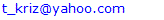Abstract

An updated review is presented to demonstrate the impact an expanding cosmos on three approaches to analyzing gravity. 1) the General Relativity based tensor method, 2) the Escape Velocity based conservation of energy method, and 3) the Force-Balanced based action-reaction method. The only common solution from all three methods is a flat space result with critical matter density ρc=3H2/8πG where H is the Hubble parameter, and G is the gravitational constant. It is also shown that the use of a Force-Balance based analysis for action-reaction, derived from a conservation of energy baseline, is analytically equivalent to that of a General Relativity tensor-based analysis for a flat space solution. Galaxy-based energy conservation and force-balanced solutions in an expanding cosmos also indicate that cosmic expansion is the unique action-based cause of a gravitational reaction at a galaxy level. Friedmann solutions from General Relativity for open and closed space curvature, however, add a speed of light term to H that violates galaxy-based conservation of energy and action-reaction force balance requirements. Thus, such curved space solutions are not physically relevant in an expanding cosmos. Evidence from Guth-Linde Inflationary Universe models is also cited to support the validity of a unique galaxy-based flat space solution.

Keywords: Gravity, Action-reaction, Newton's third law, Cosmic expansion, General Relativity, Escape velocity

Cite this paper: Thomas A. Kriz, Adam T. Kriz, An Action-Reaction Interpretation of the Interaction between Cosmic Expansion and Gravitation, International Journal of Astronomy, Vol. 7 No. 1, 2018, pp. 19-23. doi: 10.5923/j.astronomy.20180701.03.

### 1. Introduction

Traditionally, throughout human history, gravity has been seen as a natural attractive force between matter-based objects as a function of composition, size, shape, weight, and position. Physically speaking though, it is the weakest of the known forces, with about 10-38 times less strength than the “strong force” that binds the nucleus of atoms together.  The first reliable mathematical description of its attractive strength was defined by Issac Newton' in his 1687 “Principia” document,  where its attractive force was characterized as a product of the mass content of the two objects times a gravitational “constant” G, and divided by the inverse square of the distance between the objects. It can be seen, however, that although the Newton inverse square formula does not provide a clear causal basis for gravity, it does imply that the true cause of gravity is probably something that is proportional to mass of the objects. An accurate solution to gravitational attraction between objects, however, requires use of a gravitational field map that can be analyzed to obtain potential magnitude information that defines an accurate force result. At Newton's time, however, no such concept was known, so the impact of such map defined information was largely absorbed into the gravitational “constant” G. Such an analytical field map method would not be developed until the early 20th century.  It is now known that the value of the so-called “constant G” in Newton's inverse square formula actually changes with distance in the formula, especially at very short distances, but this could not be accurately measured in 1687. However, note also that, at far-field distances between objects, the Newtonian formula is remarkably accurate. 
The General Relativity paper by Einstein in 1915, [5, 6] did, however, make use of a mathematical field map analysis method that demonstrated how an observer's view of space and time was affected by a gravitational field, and gave an entirely new perspective on how gravity could impact the observed geometry of a matter object. For example, a planet in orbit around the sun, could actually be viewed as falling gravitationally. It also showed that the Newtonian formula was only accurate as a “far field” limit on a gravitational field. However, General Relativity was also matter mass based, and therefore also failed to define a clear causal basis for gravity, the same as the Newtonian formula.
Accurate field mapping analysis techniques in general were first developed early in the Twentieth century. The initial forms were graphical, such as those developed to provide electromagnetic graphical field solutions to Maxwell's Equations to aid in the design of electrical machinery in 1915 by Douglas.  Einstein's General Relativity in the same year made a giant leap forward, in that, in place of a graph, it actually made use of a mathematical model, based upon the Riemannian Geometry, that was equivalent to a set of ten simultaneous non-linear partial differential equations, which were used to obtain metric tensor solutions for gravity in a space-time geometry.  A similar mathematical approach to obtain the easier linear solutions to Maxwell's Equation set were not developed until almost 20 years later (around 1935), to support the design of RADAR systems.  However, as shown in the text by Ohanian and Ruffini,  it is also possible to develop a linear approximation of the General Relativity equation as an analog to Maxwell's Equations to get a simpler, more solvable model for some gravity problems.
The usual approach to solving a field system of partial differential equations generally involves defining the governing equation set along with boundary conditions, and then pursuing a “guess and check” process to obtain solutions.  In such a case, there generally is no substitute for a good guess. When the General Relativity equation is used as the governing equation set, however, such a process is very complex. Thus, only about a half- dozen physically relevant solutions have been found during the last hundred years.  The process can be much simplified, however, if Special Relativity is used as a constraint condition when doing tensor-based analysis.  As shown in the text by Ohanian and Ruffini, there is no relativity principle which is more general than Special Relativity.  Thus, Einstein's General Relativity is actually subservient to Special Relativity, in this regard.

### 2. General Relativity Tensor Analysis Method

Before the development of the 100” telescope on Mount Washington, observations in astronomy were limited to views inside the Milky Way galaxy, which internally appeared to have a near-static form. In 1929, use of the) 100” telescope, however, allowed astronomers to observe the expanding cosmos beyond the Milky Way. The question then is: How does the Milky Way galaxy avoid the impact of the cosmic expansion dynamic internally to have a local galaxy-based environment that seems equivalent to a near-static condition? The obvious response is that the mass of each galaxy is sufficiently large for its gravitation to balance the outward pull of cosmic expansion.
Before the discovery of the expanding cosmos in 1929 by Hubble  using the Mount Washington telescope, a primitive General Relativity model for the cosmos had already been developed. In 1922-1924, a Russian living in St. Petersburg named Alexander Friedmann [16, 17] was able to solve the Einstein General Relativity field equation assuming a simplified model of the cosmos.
The Friedmann model presumes a near-static form of the cosmos, commonly accepted at the time, and Einstein’s General Relativity equation, defined here in cgs units as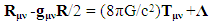(1)
where Rμν is the Ricci curvature tensor, gμν is the metric tensor, R is the Riemannian scalar curvature tensor, G is the gravitational constant, c is the speed of light, Tμν is the energy-momentum tensor of matter, and Λ is the cosmological constant. The cgs form of (1) is used here (as opposed to the Einstein convention with c=1) to enable evaluation of a solution's support for gravitational relevance, and consistency with conservation of energy and action-reaction force balance requirements in an expanding cosmos.
The Friedmann model also assumes that the sum of the rest matter in the cosmos is equal to a stable non-zero constant in a cosmos that is uniformly homogeneous and isotropic at scales larger than 100 Mpc, with a cosmological metric of form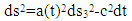(2)
where ds32 is a 3-dimensional metric that defines space curvature attributes, and a(t) defines the scale factor attributes in (1). For convenience, note that a Robertson-Walker metric is used here, rather than the awkward arguments given in the original Friedmann publications. Then, noting that the Christoffel symbols for (2) give a Ricci tensor coefficient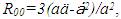and that Tμν equals matter density ρ in a perfect fluid-filled cosmos as a function of cosmic mass and volume, the form defined in (1) for the Friedmann model can then be reduced to two independent equations, one for cosmic space expansion and a second for acceleration-based space changes as a function of the curvature attribute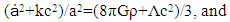(3)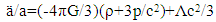(4)
where k is interpreted as a space attribute parameter (+1, 0, -1) for respectively open, flat, or closed space, ρ is the rest matter density of the cosmos, and pressure p=0. The form of (3) and (4) for the Friedmann model, respectively, when Λ=0, and ȧ2/a2=H2 is the Hubble parameter squared (only in use after the Hubble discovery of an expanding cosmos in 1929), then reduce to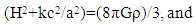(5)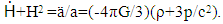(6)
With the cosmological constant Λ=0 and the curvature parameter k=0, the unique critical matter density solution for flat space in (5) then further reduces to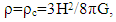(7)
which is a later mathematical result not presented in the two Friedmann papers. In fact, the Friedmann solution results were more like those given in (3) and (4) with k=+1, and k=-1 for the open and closed space solutions. It can be seen then that the term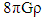in (3) and (5) indicates that total cosmic rest mass is a constant, and uniformly distributed, implying that the cosmos in the Friedmann model can be interpreted as having only one galaxy (eg; the Milky Way) in which matter is presumed to always have existed.
It can be seen that the term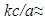(3x108m/s per million light-yrs) in (3) and (5) is equivalent to adding a speed of light change to the value of H, which is questionable even in a near static model of the cosmos because of Special Relativity constraints. In this regard, however, note that current measurements for H show that a reasonable value in an expanding cosmos is approximately 17 km/s per million light-years. [20, 21] Theoretically, such a speed of light change to H in an expanding cosmos can only occur during an ideal radiation dominated phase when there is no gravity. It will be shown in the following that such curved space solutions also violate galaxy-based conservation of energy and action-reaction force balance requirements in an expanding cosmos.

### 3. A Conservation of Energy based Escape Velocity Analysis Method

Alternatively, a simpler energy-based solution for the relation between cosmic expansion and gravity in an expanding cosmos can be found in the texts by Beiser. [20, 21], and Tipler and Llewellen . The method makes use of a galaxy-based escape velocity analysis approach that can be associated with cosmic expansion rate. Within the context of an expanding cosmos, the interaction between such expansion and gravity at each galaxy, therefore, is presumed to have an energy balanced form. Mathematically then, the energy balance for the interaction of cosmic expansion with gravity at a galaxy can be defined as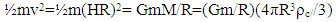(8)
where ½mv2 is the kinetic energy of the galaxy due to cosmic expansion with v as red-shift focused outward velocity, m is the mass of the galaxy, M is the total rest mass of the cosmos modeled as a spherical volume with radius R external to the galaxy, G is the gravitational constant, H=ȧ/a is the Hubble parameter where v=HR, and ρ=ρc is the critical matter density of the cosmos where expansion and gravitation are balanced. This then also yields an energy based critical matter density solution like that given in (7) that satisfies galaxy-based conservation of energy. 
As mentioned above, it is well known that current observations indicate that a reasonable value for H in an expanding cosmos is approximately 17 km/s per million light-years. [20, 21] In this regard, note that the formula in (8) can be viewed as defining a cosmic model with galaxies that satisfy cosmic conservation of energy uniquely with a flat space solution.  Quantitatively, the Friedmann curved space solutions for the General Relativity in an expanding cosmos, however, would imply that M≈0 as in an ideal radiation dominated phase, that in turn causes the form of (8) to become a null state. Thereby Friedmann curved space solutions fail to satisfy the galaxy-based conservation of energy relationship defined in (8) and must be viewed as physically irrelevant.

### 4. Newton's Third Law of Action-Reaction

Since energy can be represented as a force through a distance, the implied force on the galaxy due to cosmic expansion and the force of gravity implied by energy equation (8) then can be defined as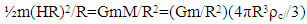(9)
which converts the galaxy-based energy balanced form in (8) to a force-based form where Newton's gravity formula, GmM/R2 serves as a measure of the far-field gravitation seen at a galaxy. It can also be seen from (8) that a gravity-based interaction (with ρ=ρc) between m and M, provides the only equal and opposite force reaction that opposes the causally based action force of cosmic expansion. This indicates then that the negative energy gravitational reaction process does not have to have the same process form as the positive action term, as is common in most cases, to fulfill the force requirements of Newton's Third law for action-reaction. Equation (9) then, due to red-shift expansion dynamic, can only reach null value when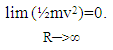(10)
Note that the energy and force balanced solutions in both (8) and (9) both imply the validity of the flat space solution in (7)  which thereby satisfies a galaxy-based conservation of energy and action-reaction force-balance requirement. Friedmann curved space solutions, however, add a speed of light kc/a term to the Hubble parameter, implying that M≈0, and cause (9) to have a null state. Such curved space solutions therefore can be seen as failing to satisfy the galaxy-based conservation of energy and action-reaction force balance requirements defined in (8) and (9).
It can also be shown that the action-reaction formula in (9) is analytically equivalent to that defined above in (5) when k=0. To see this, first note that the term m in (9) is common in all expressions separated by an equal sign, and therefore can be eliminated. Multiplying the first and last expressions defined in (9) then by a factor of 2 then yields the form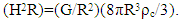(11)
Now, since the resulting term in R is also the same on both sides of the equation in (11), the term R can also be eliminated, thereby yielding the same General Relativity expression in (5) with k=0, and a flat space solution. Thus, the General Relativity analysis that defines (5) is analytically equivalent to that for an action-reaction gravitational basis defined in (9).

### 5. An Inflation based Evolutionary Scenario

The scenario described here begins with an electromagnetic energy focused process described in another paper published by the Alpha Omega group.  Such an inflation-like event is natural and needs no artificially defined form, but still yields the same inflationary impact as those found in the Guth and Linde Inflationary models. 
Initial conditions for the event assume that radiation wavelength at start is proportional to Planck Time (eg; λ∝10-45s), and initiates a radiation dominated phase that has no matter and thereby expands at light speed. At time proportional to ~1013 seconds, the radiation dominated phase ends with radiation wavelength of λ∝10-15s, thereby completing the Inflation-like process, and is followed by a three-stage decoupling event that initiates a matter dominated phase that is in accord with observed data from particle collision experiments.
It should be noted that this decoupling time event at ~1013 seconds at this point is also consistent with that found in Inflationary models. The start of the matter dominated phase then sets the stage for the balanced condition between the positive energy cosmic expansion process and the negative energy gravitation process described in (8) and the force balanced activity defined by (9). Cosmic expansion continues until the conditions define in (10) are satisfied when both cosmic expansion and gravitation no longer exist.
If Newton's Third law must always be true during the matter dominated phase in order to conserve energy, the processes in (8) and (9) involving cosmic expansion and gravitation continue until satisfying the condition defined in (10). Thus, cosmic expansion and gravitation can therefore only reach a null condition simultaneously. The open space (eg; with k=+1, ρ<ρc) and closed space (eg; with k=-1, ρ>ρc) solutions to (3) and (5) developed by Friedmann, however, add a speed of light term to H, and thereby fail to satisfy both action-reaction force as well as conservation of energy requirements.
What happens when cosmic expansion stops and gravity fails? Stars, planets, galaxies, black holes, and other material objects come completely apart, and solar-like system structures no longer exist. According to Equation (10), this occurs well after star, galaxy, and solar system formation have long ceased to exist. All stars have long ago reached old age and emit no radiation. Only a dark and cold cosmos remains full of primitive matter. Newton's Third law may still remain, but without serious impact.

### 6. Conclusions

It is shown in the foregoing that the force balance between cosmic expansion and gravitation, though different in process form, uniquely satisfies Newton's Third law with a flat space density solution of ρ=ρc=3H2/8πG. Such a flat space geometry expands forever with an expansion rate that continues to become ever smaller. Indeed, in the vast majority of cases, both the action and reaction components involving Newton's Third law do have the same energy process form, but, note that the important part of the law is the requirement that forces be balanced. It also can be seen then, in the case of cosmic expansion as an action-based force, there is no equal and opposite balancing reaction force other than that from gravitation.
It is also shown that a galaxy-based action-reaction force-balanced analysis is equivalent to a General Relativity analysis procedure with k=0 for a flat space solution. When using a Friedmann based open space-time k=+1 (with ρ<ρc ) solution, or the closed space-time k=-1 (with ρ>ρc ) solution, however, a speed of light term is added to the Hubble parameter H that violates galaxy-based requirements for conservation of energy and action-reaction force balance in an expanding cosmos.
It should also be noted that cosmic expansion is a positive energy action form and that gravitation is a negative energy reaction form. Thus, the sum of both together, per Newton's Third law, yields a net null energy condition. Both together then also constitute a necessary condition set for the existence of gravity. A corollary result to the irrelevance of a closed space solution during cosmic expansion is that a BIG CRUNCH cosmic collapse is unlikely. If ρ=ρc uniquely, a reversal of outward cosmic expansion can never occur.
It should be noted the results given here are also consistent with those from cosmic Inflationary models. [24, 25] As long as Inflation continues long enough, the state of space-time in the cosmos, viewed as an expanding 3-sphere in 4-sapce, is driven toward a flat space-time condition.  Similarly, in terms of a 2-sphere balloon model, as the balloon continues to be inflated, the flatter its surface becomes. Thus, Inflationary models generally lead to a ρ=ρc flat space solution.
As an alternative to the Guth and Linde Inflationary models, a more natural, Inflation-like model discussed above, is one which begins with initial photon wavelength proportional to Planck Time (λ10-45s). This initial surge of energy is followed by a matter free radiation dominated phase expanding at the speed of light for ~1013 seconds that ends with λ10-15s at the beginning of a matter dominated phase, same as in the Guth and Linde models. Thereby, the overall inflationary impact (eg; 1030) is also the same as that found in traditional Inflationary models.
It should be noted that some observations on type 1a supernovae  appear to give evidence of late era acceleration in the cosmic expansion rate, following the matter dominated era discussed here. There is currently ongoing study to learn more about a possible interaction.

### ACKNOWLEDGEMENTS

The authors wish to express gratitude for the encouragement and critical review received during the earlier stages of the work present here received from E.J. Bacinich, Director of the Alpha Omega Research Foundation, and other members of our group, now deceased: Prof. Behram Kursunoglu and Dr. Walter Rosenthal. Prof. Kursunoglu was the Director of the Theoretical Institute at the University of Miami (FL), and pursued graduate studies at Cambridge University under P.A.M. Dirac. Dr. Walter Rosenthal was one of the last students to pursue graduate studies in physics at the University of Berlin under Max Planck.

### References

  A. Beiser, 1995, Concepts of Modern Physics, 5th ed., McGraw Hill, New York, p 496.  I. Newton, 1687, Principia etc., British Royal Society, London.  P. Moon and D.E. Spencer, 1961, Field Theory for Engineers, D. Van Nostrand Co., Princeton, NJ.  H.C. Ohanian and R. Ruffini, 1994, Gravitation and Spacetime, 2nd ed., Norton & Co., New York and London, pp.158-163.  A. Einstein, 1915, Grundgedanken der allgemeinen Relativitätstheorie und Anwendung dieser Theorie in der Astronomie, Preussische Akademie der Wissenschaften, Sitzungsberichte.  A. Einstein, 1916, Die Grundlage der allgemeinen Relativitätstheorie, Annalen der Physik (s. 4), 49, 769–822.  J.F.H. Douglas, 1915, The reluctance of some irregular fields, AIEE Trans., 43, 1061.  H.C. Ohanian and R. Ruffini, 1994, Gravitation and Spacetime, 2nd ed., Norton & Co., New York and London, pp. 380-385.  M. Bragg, 1988, RDF1 The Location of Aircraft by Radio Methods 1935–1945, Hawkhead Publishing, London.  H.C. Ohanian and R. Ruffini, 1994, Gravitation and Spacetime, 2nd ed., Norton & Co., New York and London, pp.140-146.  P. Moon and D.E. Spencer, 1961, Field Theory for Engineers, D. Van Nostrand Co., Princeton, NJ.  S.H. Kramer, et al., 2003, Exact Solutions of Einstein's Field Equations, 2nd ed., Cambridge University Press, Cambridge.  T.A. Kriz, 2015, Half-spin particles modeled as electromagnetic resonance based micro-black-holes, American J. of Modern Phys., 4(1), 10-14.  H.C. Ohanian and R. Ruffini, 1994, Gravitation and Spacetime, 2nd ed., Norton & Co., New York and London, p.377.  P. Kupperberg, 2005, Hubble and the Big Bang, The Rosen Publishing Group, New York.  A. Friedmann, 1922, Über die Krümmung des Raumes, Z. Physik, 10(1), 377-386.  A. Friedmann, 1924, Über die Möglichkeit einer Welt mit konstanter negativer Krümmung des Raumes, Z. Physik, 21(1), 326-332.  H.C. Ohanian and R. Ruffini, 1994, Gravitation and Spacetime, 2nd ed., Norton & Co., New York and London, p.382.  H.C. Ohanian and R. Ruffini, 1994, Gravitation and Spacetime, 2nd ed., Norton & Co., New York and London, pp. 552-560.  A. Beiser, 1995, Concepts of Modern Physics, 5th ed., McGraw Hill, New York, pp. 13-14.  A. Beiser, 1995, Concepts of Modern Physics, 5th ed., McGraw Hill, New York, pp. 502-504.  P.A. Tipler and R.A. Llewellyn, 2008, Modern Physics, 5th ed., W.H. Freeman & Co, New York, pp. 662-664.  T.A. Kriz, 2017, An energy-based analysis of the quark/hadron impact on cosmic decoupling, American J. of Modern Phys., 6(6), 148-152.  A. Guth, 1996, The Inflationary Universe, Addison Wesley, New York.  H.C. Ohanian and R. Ruffini, 1994, Gravitation and Spacetime, 2nd ed., Norton & Co., New York and London, p 546.  A.G. Riess et al. 1998, Observational evidence from supernovae for an accelerating universe and a cosmological constant, AJ, 116(3), 1009-1038.